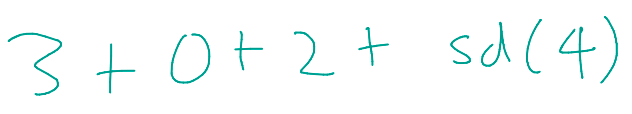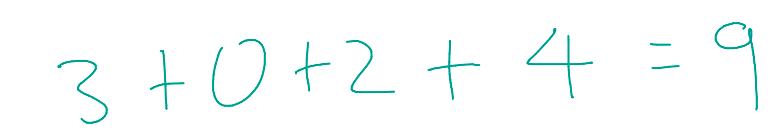# Chapter 3: Extra Examples

Now that we understand recursive calls, it'll probably be useful to walk through a couple problems in working code to see how we can apply the split strategy to different types of problems.

### Recursive Problem #3: Sum Digits

Consider any integer; we'll use 4,203 in this example.Our goal is this: how can we sum the digits of 4203? For humans, it's easy enough, but for computers, they can't just directly separate the digits; 4,203 looks roughly more like 4,000 + 200 + 00 + 3 to them. Looks like split recursion will come in handy!
Before we begin, let's define our function sd that has a parameter number, a positive integer such as 4203. sd aims to return the sum of the digits of number!
def sd(number):
#returns the sum of the digits of number!
As review, the recursive tool I call split recursion splits the problem into:
1. 1.
A solved or easily solvable problem
2. 2.
A problem that can be further split in a similar way
Our current "problem" is 4,203. We know we have to make it smaller somehow, but how can we do that?
Well, we are trying to sum up each digit of 4,203, so it probably makes sense to make 4,203 one digit smaller each time.
We can do that by dividing it by 10. Let's see what that looks like:The modulo (%) operator divides two numbers and returns their remainder.
6 % 3 = 0
8 % 3 = 2
5 % 2 = 1
4203 % 10 = 3
The floor division (//) operator divides two numbers...and throws away the remainder.
13 // 5 = 2
14 // 3 = 4
4203 // 10 = 420
Using these two operators, we can separate 4,203 into 3 and 420; this seems promising!
1. 1.
A solved or easily solvable problem: the rightmost digit of 4,203 = 3
2. 2.
A problem that can be further split in a similar way: the rest of 4,203 = 420Let's frame this into code. As a reminder, this is our recursive call:
def sd(number):
rightmost_digit = number % 10
rest_of_number = number // 10
return rightmost_digit + sd(rest_of_number)
True to our split strategy, we split 4,203 into the rightmost digit and the rest of the number; we split the rest of the number in a similar way, until it has been split into something we can immediately solve. Let's continue splitting:Seems like it's working: our problem is getting smaller!How can we split (4) any further? The answer is: we can't! And that's a good thing: we've reached our base case, when the problem has been made so small we can easily solve it.We did it! Let's look at what the base case looks like in code:
def sd(number):
if number < 10:
return number
rightmost_digit = number % 10
rest_of_number = number // 10
return rightmost_digit + sd(rest_of_number)
This is working code! If you'd like, you can run it in Python and try it out for yourself.
For a visual recap of what's going on,
sd(4203) = 3 + sd(420) #9
V
(0 + sd(42)) #6
V
( 2 + sd(4)) #6
V
4 #4

#### Recursive problem #3.5: Counting

This is more of a spin-off of the previous problem.
Every time we split the problem into two smaller problems (one solvable, one further splittable), the solvable problem was usually some value, such as the 5 pound square gold bar, or the rightmost digit in sum digits. However, we don't really care about that value when we're counting things. Instead, we just note if we find something to count, or don't do anything if it's not worth counting. The following example will help clear up what I'm talking about.
Consider any number, such as 4203. Instead of summing the digits, this time, we want to count the number of digits in the number. To you, a human being, you can glance at at it and instantly realize it's 4 digits. However, as I said earlier, the computer can't do that easily. So, let's use recursion! We can splitthe problem in the same way as before, but this time, we don't care about the actual value of the number, just that we can count that there's a number there.
Sum digits provides us with a great framework to work with; the base case is mostly there, and the recursive call is mostly set up for us.
def count(number):
if number < 10:
return ___
rest_of_number = number // 10
return ___ + count(rest_of_number)
What's a number we could replace the blank in the recursive call with? Well, each time count(number) is called, we count ONE digit. So, it makes sense that we could just add one every time the recursive call happens. At the same time, if we reach the base case, we only have ONE digit left, so it makes sense to replace the blank in the base case with 1.
def count(number):
if number < 10:
return 1
rest_of_number = number // 10
return 1 + count(rest_of_number)
That's counting! Surprisingly enough, you'll encounter counting a lot, as it's pretty useful.

### Recursive Problem #4: Factorial

The factorial of a number n is defined as the product of n with every positive integer before it. It is written as "n!"
For example, 5! = 5 x 4 x 3 x 2 x 1
Written generally, n! = n x (n-1) x (n-2) x (n-3) x ... x 1
If we were to write a recursive function to solve this, our split strategy will definitely come in handy.
Considering 5!, we know we want to make that problem smaller. So, let's try to relate it to 4!
5! = 5 x 4 x 3 x 2 x 1
4! = 4 x 3 x 2 x 1
It should be clear that 5! = 5 x 4!
Interestingly, it seems we've split "5!" into:
• an easily solvable problem, 5
and
• a smaller problem that can be split in a similar way, 4!
Consider that for any number n:
n! = n x (n-1)!
1. 1.
n is a solved problem, because we know the value of n
2. 2.
(n-1)! is a smaller problem that can be split in a similar way as n!
def factorial(n):
return n * factorial(n - 1)
This is our recursive call! Hopefully, this should be very similar to the other examples. Notice that I split the problem into two problems multiplied by each other, while our previous example used addition. No matter the way you split the problem, the splitting strategy should work!
Of course, we have to tell the function when to stop.
For what value of n can we immediately solve "n!"?
1! = 1, which is easily solvable. Let's see that in code:
def factorial(n):
if n == 1:
return 1
return n * factorial(n - 1)
As a reminder, this is working code that you can run in Python if you wish. For a visual recap,
factorial(5) = 5 * factorial(4) #120
V
(4 * factorial(3)) #24
V
(3 * factorial(2)) #6
V
(2 * factorial(1)) #2
V
1

### Phew!

If you've made it this far, congratulations! You probably have a good idea of what a few recursive tools look like now. However, even the sharpest sword is useless in the hands of an inexperienced warrior. You'll need to practice to get a good grasp of recursive thinking. In the next chapter, you'll find several exercises that use the ideas from this chapter: please try your best at them! Practice is really really REALLY important.